Evolutionary Algorithm-based Approach for Multi-Objective Optimization of a Complex Reliability System

Anuj Kumar1,*, Sangeeta Pant2, Mangey Ram3, 4 and Akshay Kumar5

1Department of Mathematics, University of Petroleum and Energy Studies, Dehradun, India
2School of Engineering and Computing, Dev Bhoomi Uttarakhand University, Dehradun, India
3Department of Mathematics, Computer Science & Engineering, Graphic Era Deemed to be University, Dehradun, India
4Institute of Advanced Manufacturing Technologies, Peter the Great St. Petersburg Polytechnic University, Saint Petersburg 195251, Russia
5Department of Mathematics, Graphic Era Hill University, Dehradun, India
E-mail: anuj4march@gmail.com
*Corresponding Author

Received 11 November 2022; Accepted 31 January 2023; Publication 11 April 2023

## Abstract

Dealing with the conflicting objectives in reliability analysis of complex engineering systems is always a challenging task. Here, we have taken two conflicting objectives namely reducing cost and increasing the reliability of a complex reliability system named life support system in space capsule (LS3C) into consideration. A novel multi-objective evolutionary algorithm named MOPSO-CD has been employed to get various Pareto Optimal Fronts (POFs) in accordance with different parameter tuning. The simulation results so obtained provide a wide range of varieties of POFs to decision maker (DM).

Keywords: Reliability, cost, multi-objective optimization problem (MOOP), evolutionary algorithm, pareto optimal fronts (POFs), particle swarm optimization incorporating crowding distance (MOPSO-CD).

## 1 Introduction

In today’s industrial scenario, DM are bound to take decisions for various multiple conflicting objectives in uncertain environments for optimal RAMS required industrial products . Evolutionary algorithms usually designed to find a better approximation to the complete Pareto-optimal front (POF), which then allows the DM to decide, among many available alternatives. The last two decades have witnessed enormous growth in the field of evolutionary algorithms developed either for single objective or for multi-objective problems . The reason behind the enormous growth in the development of EA is the nonlinear, non-differentiable, discontinuous, multi-modal objective functions correspond to a real-life industrial problem with almost no idea about their behaviour [10, 11]. Hence, traditional optimization methods fail to handle the complexities associate with complex real life industrial optimization problems [12, 13].

In this work, we present the framework of the implementation of MOPSO-CD for a complex bi-objective reliability cost optimization named LS3C.

Section 2 provides the material and methods and consist of a brief description of MOPSO-CD and the considered MOOP problem associated with a complex system named LS3C. Section 3 present the results and discussion followed by the conclusion in Section 4.

## 2 Material & Method

### 2.1 MOPSO-CD

Multi-objective version of the most popular algorithm, particle swarm optimization is named as MOPSO, which is a swarm-based technique [14, 15].

Here, a particular version of MOPSO named as MOPSO-CD introduced by Raquel and Naval  has been presented for getting the POFs of the bi-objective reliability cost optimization problem associated with LS3C. The flowchart of MOPSO-CD is depicted in Figure 1. More details about the same can be find in [7, 16].Figure 1 The flow chart of MOPSO-CD.

### 2.2 LS3C

The continuous non-linear programming problem of LS3C is NP-hard in nature . It contains four subsystems, which are connected in series, parallel and mixed configurations as represented in its block diagram (Figure 2). This reliability optimization problem is to obtain the minimum system cost and maximum system reliability simultaneously.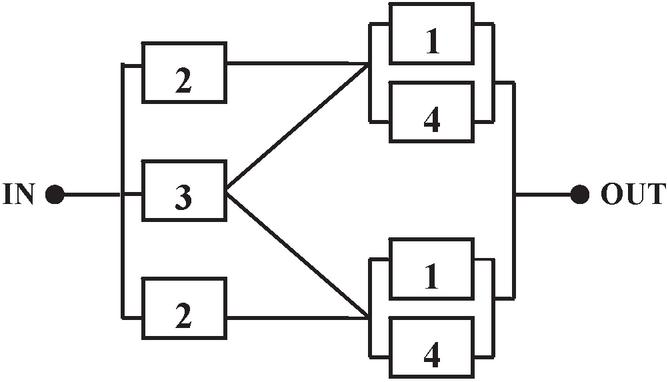Figure 2 Block diagram of LS3C.

The above-mentioned system has subcomponents having component reliability $r⁢es$, $s=1⁢…⁢4$. The reliability and the cost of LS3C are given by

 $RL⁢S⁢3⁢C$ $=1-r⁢e3⁢[(1-r⁢e1)⁢(1-r⁢e4)]2$ $-(1-r⁢e3)⁢[1-r⁢e2⁢{1-(1-r⁢e1)⁢(1-r⁢e4)}]2$ $CL⁢S⁢3⁢C$ $=2⁢L1⁢r⁢e1α1+2⁢L2⁢r⁢e2α2+L3⁢r⁢e3α3+2⁢L4⁢r⁢e4α4$

where, $L1,L2=100,L3=200,L4=150$, $αj=0.6,j=1⁢…⁢4$.

Thus, the problem is to find decision variables (component reliability) $r⁢es$, $s=1,2,3,4$ which minimize the overall system cost and its unreliability and satisfy the imposed constraints, i.e.

Find $(r⁢e1,r⁢e2,r⁢e3,r⁢e4)$ which minimize $(QL⁢S⁢3⁢C,CL⁢S⁢3⁢C)$ subject to

 $0.5≤r⁢es≤1,s=1⁢…⁢4.$

## 3 Numerical Simulation and Discussion

In this section, we analyzed the results achieved by applying MOPSO-CD correspond to different parameters setup for LS3C. Initially, we have started with constant inertia weight, subsequently the POFs corresponds to different mutation probabilities and acceleration coefficients have been evaluated.Figure 3 POF for LS3C for $w=0.3$, $c1=c2=1.0$ and $Pmut=0.3$.Figure 4 POF for LS3C for $w=0.3$, $c1=c2=1.0$ and $Pmut=0.6$.

After performing several computation tests, various POFs obtain have been presented by the help of various figures (Figures 3 to 26). For each computation test, the swarm size is taken as 200 with 400 maximum generations with an archive size of 200. Further, we have investigated the impression of different parameter settings on the nature and behavior of POF. The following investigation has been performed:

(i) The POFs corresponding to different inertia weights ($w=0.3$, 0.6, 0.9, 1.20) have been evaluated.Figure 5 POF for LS3C for $w=0.3$, $c1=c2=1.0$ and $Pmut=0.9$.Figure 6 POF for LS3C for $w=0.3$, $c1=c2=2.0$ and $Pmut=0.3$.Figure 7 POF for LS3C for $w=0.3$, $c1=c2=2.0$ and $Pmut=0.6$.Figure 8 POF for LS3C for $w=0.3$, $c1=c2=2.0$ and $Pmut=0.9$.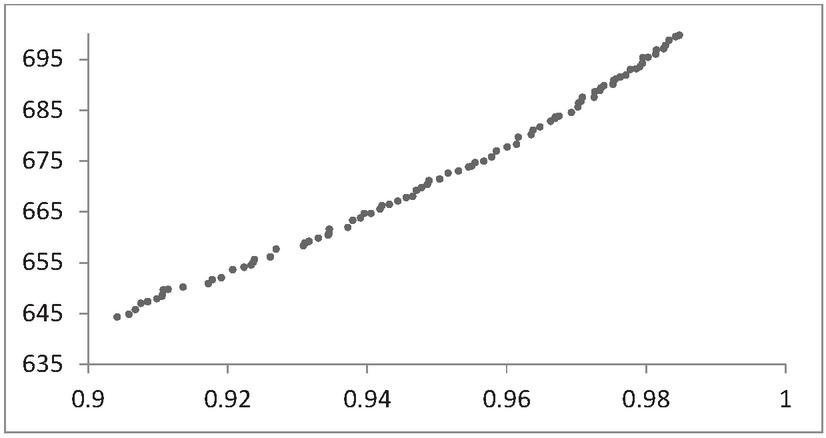Figure 9 POF for LS3C for $w=0.6$, $c1=c2=1.0$ and $Pmut=0.3$.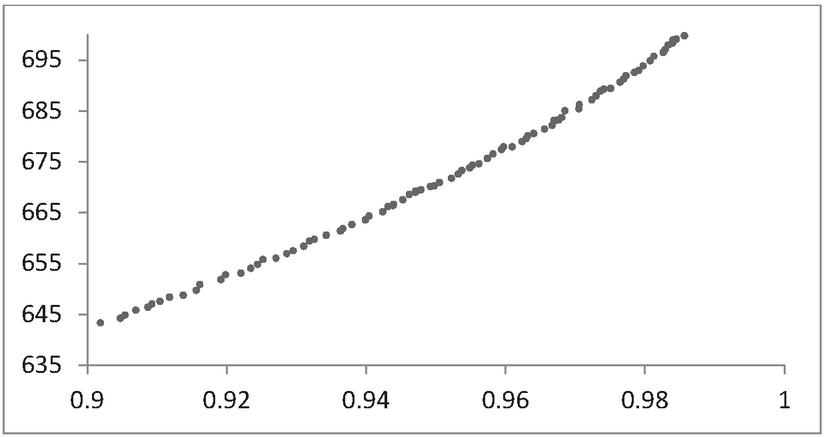Figure 10 POF for LS3C for $w=0.6$, $c1=c2=1.0$ and $Pmut=0.6$.Figure 11 POF for LS3C for $w=0.6$, $c1=c2=1.0$ and $Pmut=0.9$.Figure 12 POF for LS3C for $w=0.6$, $c1=c2=2.0$ and $Pmut=0.3$.Figure 13 POF for LS3C for $w=0.6$, $c1=c2=2.0$ and $Pmut=0.6$.Figure 14 POF for LS3C for $w=0.6$, $c1=c2=2.0$ and $Pmut=0.9$.Figure 15 POF for LS3C for $w=0.9$, $c1=c2=1.0$ and $Pmut=0.3$.Figure 16 POF for LS3C for $w=0.9$, $c1=c2=1.0$ and $Pmut=0.6$.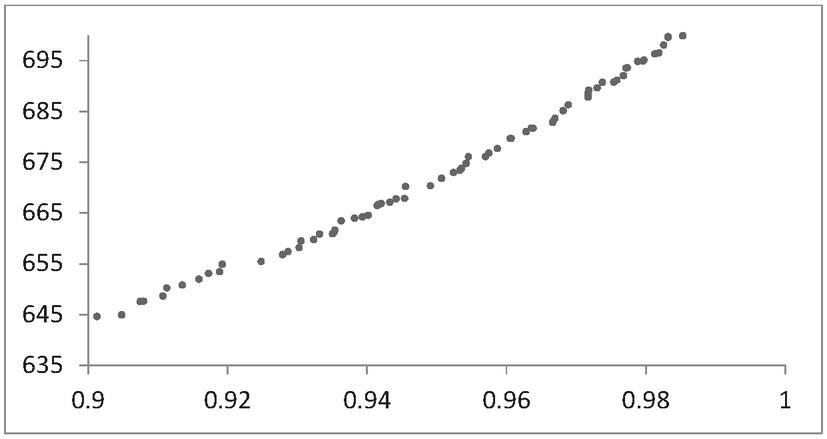Figure 17 POF for LS3C for $w=0.9$, $c1=c2=1.0$ and $Pmut=0.9$.Figure 18 POF for LS3C for $w=0.9$, $c1=c2=2.0$ and $Pmut=0.3$.Figure 19 POF for LS3C for $w=0.9$, $c1=c2=2.0$ and $Pmut=0.6$.Figure 20 POF for LS3C for $w=$$0.9$, $c1=c2=2.0$ and $Pmut=0.9$.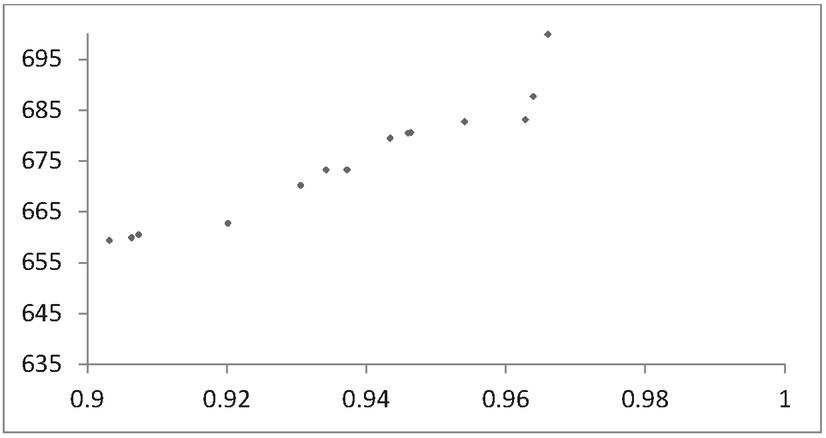Figure 21 POF for LS3C for $w=1.2$, $c1=c2=1.0$ and $Pmut=0.3$.Figure 22 POF for LS3C for $w=1.2$, $c1=c2=1.0$ and $Pmut=0.6$.Figure 23 POF for LS3C for $w=1.2$, $c1=c2=1.0$ and $Pmut=0.9$.Figure 24 POF for LS3C for $w=1.2$, $c1=c2=1.0$ and $Pmut=0.3$.Figure 25 POF for LS3C for $w=1.2$, $c1=c2=1.0$ and $Pmut=0.6$.Figure 26 POF for LS3C for $w=1.2$, $c1=c2=2.0$ and $Pmut=0.9$.

(ii) The acceleration coefficients (ACs) are either fixed at 1.0 or at 2.0 for each of the above-mentioned inertia weights.

(iii) For the above-mentioned combination the mutation probability (MP) has been tested for the values 0.3, 0.6 and 0.9.

By fixing $w$ at 0.3 and $c1$, $c2$ at 1.0 and varying the mutation probability, we get almost same POFs with good convergence as shown in Figures 35. Further, POFs loses their uniformity on fixing the acceleration coefficients at 2.0 for the same mutation probability and inertia weight. These POFs are presented in Figures 6 to 8. When we set $w$ at 0.6 and AC at 1.0, we get diverse solutions for the different mutation probabilities. But on setting the value of acceleration coefficients at 2.0, the POFs lose uniformity and hence diverges. These POFs are reported in Figure 9 to 14. Similar results have been obtained for $w=0.9$ and $w=1.20$, which are reported through Figures 1526. After that DM can adopt a decision making technique for choosing the best available alternatives of his/her choice .

## 4 Conclusion

In this article, an evolutionary algorithm named MOPSO-CD has been applied on a complex reliability multi-objective optimization problem named LS3C for obtaining various POFs correspond to different parameter settings. With the help of these POFs one can conclude that the implemented evolutionary algorithm provides well-distributed pareto optimal points from which DM can choose a particular solution of his/her choice. Further, it has been observed that the mutation probability, inertia weight along with acceleration coefficients influencing the resultant POFs.

## References

 M.S. Chern, “On the computational complexity of reliability redundancy allocation in a series system,” Operations Research Letters, vol. 11, no. 5, pp. 309–315, 1992.

 A. Kumar, S. Pant, S., M. Ram & G. Negi, “Multi-Objective Optimization of Complex Bridge Network by MOPSO-CD”, In 2022 International Conference on Advances in Computing, Communication and Materials (ICACCM), (pp. 1–6). IEEE, 2022.

 S. Chaube et al. “Time-dependent conflicting bifuzzy set and its applications in reliability evaluation,” Advanced Mathematical Techniques in Engineering Sciences. 2018 May 4:111–28.

 A. D. Salazar, C. M. Rocco and B. J. Galvan, “Optimization of constrained multiple-objective reliability problems using evolutionary algorithms”, Reliability Engineering and System Safety, Vol. 91, pp. 10571070, 2006.

 H. Garg, M. Rani, S. P. Sharma, Y Vishwakarma, “Intuitionistic fuzzy optimization technique for solving multi-objective reliability optimization problems in interval environment”, Expert Systems with Applications, vol. 41, no. 7, pp. 3157–3167, 2014.

 H. Yin et al. “Multi-objective crashworthiness optimization design of functionally graded foam-filled tapered tube based on dynamic ensemble meta model”, Materials and Design, vol. 55, pp. 747–757, 2014.

 A. Kumar, S. Pant, M. Ram, “Gray wolf optimizer approach to the reliability-cost optimization of residual heat removal system of a nuclear power plant safety system,” Quality and Reliability Engineering International. 2019 Nov;35(7):2228–39.

 A. Kishore, S. P. Yadav, S. Kumar, “Application of a Multi-objective Genetic Algorithm to Solve Reliability Optimization Problem”, in the proc. of IEEE International conference on computational intelligence and multi-media applications, pp. 458–462, 2007.

 J. Zhao, Z. Liu, M. Dao, “Reliability optimization using multi objective ant colony system approaches”, Reliability Engineering & System Safety, vol. 92, no. 1, pp. 109–120, 2007.

 A. Kumar, S. Pant, M. Ram, S. B. Singh, “On solving complex reliability optimization problem using multi-objective particle swarm optimization,” Mathematics Applied to Engineering 2017 Jan 1 (pp. 115–131). Academic Press.

 N. Uniyal et al., “An overview of few nature inspired optimization techniques and its reliability applications,” International Journal of Mathematical, Engineering and Management Sciences; 5(4):732, 2020.

 A. Kumar, S. Pant, M. Ram, O Yadav, editors, “Meta-heuristic Optimization Techniques: Applications in Engineering,” Walter de Gruyter GmbH & Co KG; 2022 Jan 19.

 H. K. Sharma, A. Kumar, S. Pant, M. Ram, “Artificial Intelligence, Blockchain and IoT for Smart Healthcare,” in Artificial Intelligence, Blockchain and IoT for Smart Healthcare, River Publishers, 2022.

 J. Kennedy and R. Eberhart, “Particle swarm optimization,” in the proc. of IEEE International Conference on Neural Networks, pp. 1942–1948, 1995.

 R. Eberhart and J. Kennedy, “A new optimizer using particle swarm theory,” in the proc. of the Sixth International Symposium on Micro Machine and Human Science, pp. 39–43, 1995.

 X. Hu, Y. Shi, and R. C. Eberhart, Recent advances in particle swarm, in the Proc. of IEEE Congress on Evolutionary Computation, pp. 90–97, 2004.

 C. A. Coello Coello, G. T. Pulido, and M. S. Lechuga, “Handling multiple objectives with particle swarm optimization”, IEEE Transactions on Evolutionary Computation, vol. 8, no. 3, pp. 256279, 2004.

 FA Tilman, C. Hwang, L. Fan, K. C. Lal, Optimal Reliability of a Complex System, IEEE Transactions on Reliability, vol. R-19, no. 3, pp. 95–100, 1970.

 C.M. Rocco, A.J. Miller, J.A. Moreno, and N. Carrasquero, “A cellular evolutionary approach applied to reliability optimization of complex systems”, in the Proc. of the Annual Reliability and Maintainability Symposium, pp. 210–215, 2000.

 A. Kumar, G. Negi, S. Pant, M. Ram, S.C. Dimri, “Availability-Cost Optimization of Butter Oil Processing System by Using Nature Inspired Optimization Algorithms,” Reliability: Theory & Applications. 2021(SI 2 (64)):188–200.

 J. Safari, J. “Multi-objective reliability optimization of series-parallel systems with a choice of redundancy strategies”, Reliability Engineering & System Safety, 108, 10–20, 2012.

 T. L. Saaty, “How to make a decision: the analytic hierarchy process”, European journal of operational research, 48(1), 9–26, 1990.

 A. Kumar, P. Garg, S. Pant, M. Ram, A. Kumar, “Multi-criteria decision-making techniques for complex decision making problems” Journal|MESA. 13(3), 791–803, 2020.

 S. Guangyong, L. Guangyao, G. Zhihui, C. Xiangyang, Y. Xujing, L. Qing, “Multiobjective robust optimization method for drawbead design in sheet metal forming,” Materials & Design, vol. 31, no. 4, pp. 1917–1929, 2010.

 A. Kumar, S. Pant, “Analytical hierarchy process for sustainable agriculture: An overview”, MethodsX, 101954, 2023.

 S.S. Rawat et al. “A State-of-the-Art Survey on Analytical Hierarchy Process Applications in Sustainable Development”, Int. J. Math. Eng. Manag. Serv. 7, 883–917, 2022.

## BiographiesAnuj Kumar received his Master’s and doctorate degree in Mathematics from G. B. Pant University of Agriculture and Technology, Pantnagar, India. Currently, he is working as an Associate Professor of Mathematics at University of Petroleum and Energy Studies, Dehradun, India. His current area of interest is reliability analysis, nature-inspired optimization and Multi-criteria decision-making (MCDM). He has published more than 50 research articles in journals of national/international repute and authored/edited 04 books in his area of interest. In addition, he is instrumental in various other research related activities like editing/reviewing for various reputed journals, organizing/participating in conferences.Sangeeta Pant received her Ph.D. degree with major in Mathematics and minor in Computer Science from G. B. Pant University of Agriculture and Technology, Pantnagar, India in year 2011. She has been working as an Associate Professor in School of Engineering and Computing at Dev Bhoomi Uttarakhand University, Dehradun. Prior to it she was associated with University of Petroleum and Energy Studies, Dehradun, India. She taught several core courses in pure and applied mathematics at undergraduate and postgraduate levels. Her current area of interest is nature-inspired optimization techniques and Multi-criteria decision-making (MCDM). She has published more than 45 research articles in journals of national/international repute and authored/edited 04 books in his area of interest.Mangey Ram received the Ph.D. degree major in Mathematics and minor in Computer Science from G. B. Pant University of Agriculture and Technology, Pantnagar, India in 2008. He has been a Faculty Member for around thirteen years and has taught several core courses in pure and applied mathematics at undergraduate, postgraduate, and doctorate levels. He is currently the Research Professor at Graphic Era (Deemed to be University), Dehradun, India & Visiting Professor at Peter the Great St. Petersburg Polytechnic University, Saint Petersburg, Russia. Before joining the Graphic Era, he was a Deputy Manager (Probationary Officer) with Syndicate Bank for a short period. He is Editor-in-Chief of International Journal of Mathematical, Engineering and Management Sciences; Journal of Reliability and Statistical Studies; Journal of Graphic Era University; Series Editor of six Book Series with Elsevier, CRC Press-A Taylor and Frances Group, Walter De Gruyter Publisher Germany, River Publisher and the Guest Editor & Associate Editor with various journals. He has published 300 plus publications (journal articles/books/book chapters/conference articles) in IEEE, Taylor & Francis, Springer Nature, Elsevier, Emerald, World Scientific and many other national and international journals and conferences. Also, he has published more than 55 books (authored/edited) with international publishers like Elsevier, Springer Nature, CRC Press-A Taylor and Frances Group, Walter De Gruyter Publisher Germany, River Publisher. His fields of research are reliability theory and applied mathematics. Dr. Ram is a Senior Member of the IEEE, Senior Life Member of Operational Research Society of India, Society for Reliability Engineering, Quality and Operations Management in India, Indian Society of Industrial and Applied Mathematics, He has been a member of the organizing committee of a number of international and national conferences, seminars, and workshops. He has been conferred with “Young Scientist Award” by the Uttarakhand State Council for Science and Technology, Dehradun, in 2009. He has been awarded the “Best Faculty Award” in 2011; “Research Excellence Award” in 2015; “Outstanding Researcher Award” in 2018 for his significant contribution in academics and research at Graphic Era Deemed to be University, Dehradun, India. Recently, he has been received the “Excellence in Research of the Year-2021 Award” by the Honourable Chief Minister of Uttarakhand State, India.Akshay Kumar received the B.Sc. and M.Sc. degree in science from Chaudhary Charan Singh University, Meerut, India, in 2010 and 2012, and the Ph.D. degree major in Mathematics and minor in Computer science from G. B. Pant University of Agriculture and Technology, Pantnagar, India, in 2017. He is currently working as an Assistant Professor in the Department of Mathematics, Graphic Era Hill University, Dehradun in India. He has more than 5-year teaching experience. He is a regular Reviewer for more than 35 international journals including Elsevier, Springer, Emerald, MDPI, Cambridge University Press, World Scientific, River Publisher, IGI Global Publisher and many other publishers. He has published 60 plus research papers in Communications in Statistics – Simulation and Computation., Emerald, Inderscience, International Journal of Mathematical, Engineering and Management Sciences, IGI Global Publisher, MDPI, World Scientific, Wiley Publishers, Cambridge Scientific Publishers, Springer nature, Multimedia Tools and Applications and many other national and international journals of repute and presented his works at national and international conferences. Also, he has published 2 books (edited) with international publishers like River Publisher and IGI Global Publisher. His fields of research are reliability theory, fuzzy reliability, signature reliability and applied Mathematics.